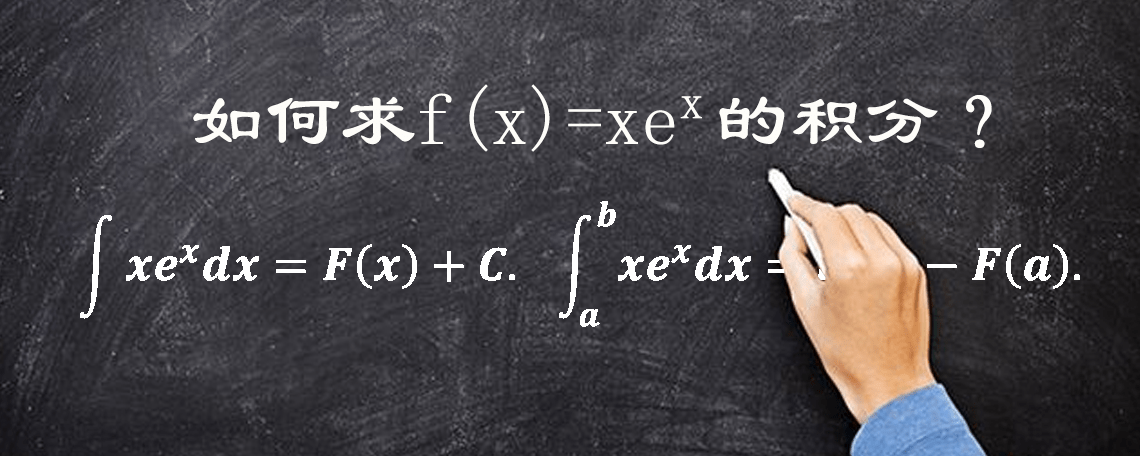# 原创 求一下这个简单的积分，它竟然涵盖积分的两大方法，解出来很有意义

xe^x的积分包括不定积分和定积分。不定积分求的是函数f(x)=xe^x的原函数，可以记为F(x)+C，它是一个函数系统。而定积分求的是曲线f(x)=xe^x与x轴在区间[a,b]上，与x=a,和x=b围成的图形面积。

xe^x的积分包括不定积分和定积分。不定积分求的是函数f(x)=xe^x的原函数，可以记为F(x)+C，它是一个函数系统。而定积分求的是曲线f(x)=xe^x与x轴在区间[a,b]上，与x=a,和x=b围成的图形面积。1、凑微分，就是把e^xdx转化成de^x。即∫xe^xdx=∫xde^x。凑微分是最常用的积分方法，一定要掌握好。它的原理是微分的逆过程，即根据de^x=e^xdx，就有e^xdx=de^x. 不仅要掌握，而且要熟练常用的凑微分公式。比如cosxdx=dsinx, (secx)^2dx=dtanx, dx/x=dlnx等。

2、分部积分法，就是积分等于被积函数与微分变量的积减去被积函数和微分变量交换位置后的积分，即∫xde^x=xe^x-∫e^xdx. 虽然分部积分法有公式，不过如果能够用自己的语言把公式描述出来，运用上自然就不会有什么障碍了。因此老黄经常鼓励学生，要学会用自己的语言去描述定义、定理、法则和公式等。

∫xe^xdx=∫xde^x=xe^x-∫e^xdx=xe^x-e^x+C=(x-1)e^x+C=F(x)+C.(最后的表示法是为了后面描述的方便)